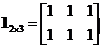## Matrix One

In some application such as regression , we need to concatenate the data matrix with vector one. A general form of vector one is matrix one , that is a matrix whose entries are all ones.

Matrix one is fully determined by its size .

Example:This simple interactive program below will produce matrix one at different sizes. This program is designed to confirm your basic understanding about matrix one.

Max Row = Max Column =

See Also : Matrix Zero , Matrix Identity